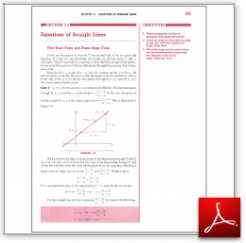Algebra Math Book - Introductory Algebra Thank you for your continual support.

 The power of mathematics rests in the logic of thinking.ID: Sec7-4
Description: Two-Point Form and Point-Slope Form-Slope-Intercept Form
Price: 1.99

# Algebra Math book - Introductory Algebra - Chapter 7 - Section 4 - Equations of Straight Lines

## Section 7.4 - Objectives

5.  Write an equation of a line in two-point form given two points.

6.  Given the slope of a line and one point on the line, write the equation in point-slope form.

7.  Given the slope and the point where the line intersects the y-axis, write the equation of the line in slope-intercept form.

Navigate to
Previous Section or Next Section
or Chapter 7 Details or Other Chapters

This section of my algebra math book, Introductory Algebra, also includes in the download:

• Cover Sheet